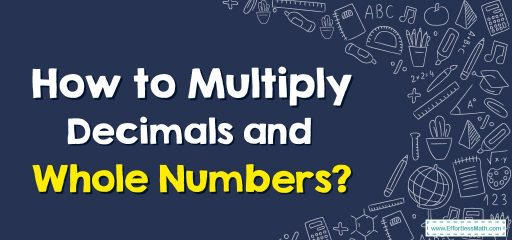# How to Multiply Decimals and Whole Numbers?

Multiplying decimals is like multiplying whole numbers, but with one difference! In this step-by-step guide, you learn how to multiply decimals and whole numbers.Multiplying decimals is like multiplying whole numbers, except for the placement of the decimal point in the product.

## A step-by-step guide to multiplying decimals and whole numbers

Multiplying decimals with whole numbers is like multiplying whole numbers, only the difference is in the placement of the decimal point. The following steps can be followed to multiply decimals with whole numbers:

• Step 1: First, ignore the decimal point and multiply the two numbers normally.
• Step 2: After multiplication, count the number of decimal places in the decimal number. The product will have the same number of decimal places.
• Step 3: Place the decimal point in the obtained product following step $$2$$.

### Multiplying Decimals and Whole Numbers – Example 1:

Find the product of $$2.31 × 12$$.

Solution:

## Find each product.

1. $$\color{blue}{17×18.68}$$
2. $$\color{blue}{1.694×4265}$$
3. $$\color{blue}{567×0.076}$$
4. $$\color{blue}{15.799×157}$$
1. $$\color{blue}{317.56}$$
2. $$\color{blue}{7224.91}$$
3. $$\color{blue}{43.092}$$
4. $$\color{blue}{2480.443}$$

### What people say about "How to Multiply Decimals and Whole Numbers? - Effortless Math: We Help Students Learn to LOVE Mathematics"?

No one replied yet.

X
30% OFF

Limited time only!

Save Over 30%

SAVE $5 It was$16.99 now it is \$11.99